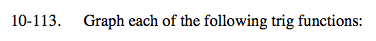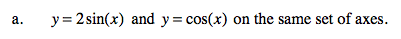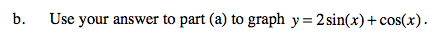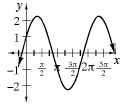### Home > A2C > Chapter 10 > Lesson 10.2.4 > Problem10-113

10-113.
1. Graph each of the following trig functions: Homework Help ✎

1. y = 2sin(x) and y = cos(x) on the same set of axes.

2. Use your answer to part (a) to graph y = 2sin(x) + cos(x).Use the input bar below to graph each trig function.Add the corresponding y-coordinates to get the graph of the sum of two functions.